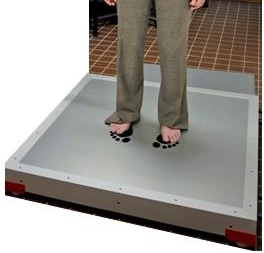# Difference between revisions of "Balance Board"

ManualsBalance Board

A balance board(force platform) is commonly used in motor control labs and neurologic clinics. They essentially consist of a set of finely calibrated scales, measuring mechanical forces. The pattern of forces can be used to derive body position, at a high spatial and temporal resolution.

## Features

There are several boards specified up to 1M2 and 0,5M2 with a sub-millimeter spatial resolution and a 200 hz temporal resolution.

A typical balance board records at a high spatial and temporal resolution. Regarding spatial resolution: a platform can detect changes from a few sub-millimeters (freezing) to at least 500 centimeter (forward or backward steps). The platform records reliably over four vertical forces. Regarding temporal resolution: the signals are typically sampled at 200 Hz. Even though the system allows even higher sampling rates, in practice 100 Hz is sufficient.

The pressure sensors derive directly from the wii balance board. Each sensor has a maximum pressure of 120Kg. In-house electronics is build to get a clean amplification from the sensors. A National instruments card, USB-6221, takes care of the A/D conversion and connects with usb to a pc. The force plate can be integrated into existing systems for stimulus presentation and for recording bodily signals such as EEG, EMG and heart rate. In practice, this means that the systems are time-locked within millisecond accuracy.

## Usage

### Neurobs Presentation

``` 1 sub runtrials_national begin
2
3 	# The dio_device will setup NI-DAQmx device number 1 "Dev1"
4 	dio_device card = new dio_device(ni_dio_device, 1, 0 );
5 	#int id = card.acquire_analog_input( "MyVoltageOutTask" );
6 	int id1 = card.acquire_analog_input( "ForceMeasurement,Voltage_0" );
7 	int id2 = card.acquire_analog_input( "ForceMeasurement,Voltage_1" );
8 	int id3 = card.acquire_analog_input( "ForceMeasurement,Voltage_2" );
9 	int id4 = card.acquire_analog_input( "ForceMeasurement,Voltage_3" );
10 	count_old = response_manager.total_response_count();
11 	loop
12 	until false
13 	begin
14 		if response_manager.total_response_count() > count_old then
15 			count_old = response_manager.total_response_count();
16 			calibrate_board = true;
17 		end;
18
19 		message_scale = round(round(card.read_analog( id1, 1000.0 ),6) * 1000.0, 0);
20 		message_scale = round(round(card.read_analog( id2, 1000.0 ),6) * 1000.0, 0);
21 		message_scale = round(round(card.read_analog( id3, 1000.0 ),6) * 1000.0, 0);
22 		message_scale = round(round(card.read_analog( id4, 1000.0 ),6) * 1000.0, 0);
23
24 		if calibrate_board then
25 			zero_scale_left_up    = message_scale[left_up];
26 			zero_scale_left_down  = message_scale[left_down];
27 			zero_scale_right_up   = message_scale[right_up];
28 			zero_scale_right_down = message_scale[right_down];
29 			calibrate_board = false;
30 		end;
31
32 message_scale[left_up]    = message_scale[left_up] - zero_scale_left_up;
33 		message_scale[left_down]  = message_scale[left_down] - zero_scale_left_down;
34 		message_scale[right_up]   = message_scale[right_up] - zero_scale_right_up;
35 		message_scale[right_down] = message_scale[right_down] - zero_scale_right_down;
36 		t_scale11.set_caption(string(message_scale[left_up]));
37 		t_scale11.redraw();
38 		t_scale22.set_caption(string(message_scale[left_down]));
39 		t_scale22.redraw();
40 		t_scale33.set_caption(string(message_scale[right_up]));
41 		t_scale33.redraw();
42 		t_scale44.set_caption(string(message_scale[right_down]));
43 		t_scale44.redraw();
44
45 		pos_dot_x = (message_scale[right_up] + message_scale[right_down]) - message_scale[left_up] + message_scale[left_down]);
46 		pos_dot_y = (message_scale[left_up] + message_scale[right_up]) - message_scale[left_down] + message_scale[right_down]);
47 		p_balance.add_part( balance_pos, (pos_dot_x * 1.0), (pos_dot_y * 1.0));
48 		t_coord.set_caption(string(pos_dot_x)+","+string(pos_dot_y));
49 		t_coord.redraw();
50
51 		p_balance.present();
52 		p_balance.remove_part( 8 );
53 	end;
54 	card.release_analog_input( id1 );
55 	card.release_analog_input( id2 );
56 	card.release_analog_input( id3 );
57 	card.release_analog_input( id4 );
58 end;
```

### Python

``` 1 from PyDAQmx import Task
2 from PyDAQmx.DAQmxConstants import *
3 from PyDAQmx.DAQmxTypes import *
4 import numpy
5 import msvcrt
6 import time
7
8 times = []
9 try :
10     freq = 100.0 # Hz
11     numinputs = 4
12
15     timer= time.clock()
16     running = True
17
18     data = numpy.zeros((numinputs,), dtype=numpy.float64)
19
20     #DAQmx Configure Code
21     analog_input.CreateAIVoltageChan("Dev1/ai0:%i" % (numinputs - 1), None, DAQmx_Val_RSE, -10.0,10.0,DAQmx_Val_Volts,None)
22     #analog_input.CfgInputBuffer(0)
23     #analog_input.CfgSampClkTiming("",freq,DAQmx_Val_Rising,DAQmx_Val_ContSamps,1000)
24
26     datalist = []
27     while running:
28         #DAQmx Start Code
29
30
31
34         ser.write('S')
36
37         time.sleep((1 / freq) - (time.clock()- timeBeforeRead))
38
40
41
42         if msvcrt.kbhit():
43             running = False
44
45
46 finally :
47     print "Stop"
48     t = numpy.array(times)
49     deviation = numpy.mean(abs(t - numpy.mean(t)))
50     maxdeviation  = max(abs(t-numpy.mean(t)))
51
52     print numpy.mean(t), deviation , maxdeviation
53
54